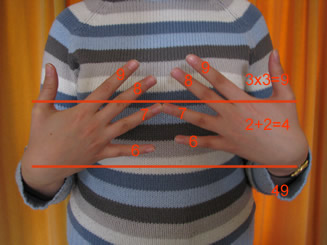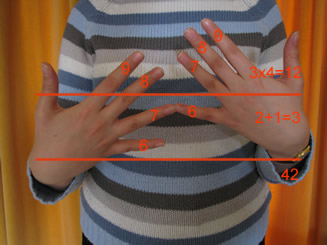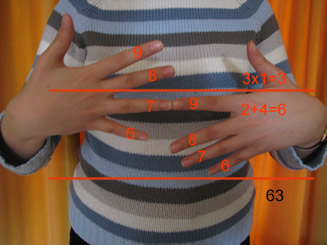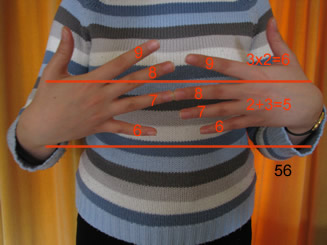#### You may also like### Adding All Nine

Make a set of numbers that use all the digits from 1 to 9, once and once only. Add them up. The result is divisible by 9. Add each of the digits in the new number. What is their sum? Now try some other possibilities for yourself!### Double Digit

Choose two digits and arrange them to make two double-digit numbers. Now add your double-digit numbers. Now add your single digit numbers. Divide your double-digit answer by your single-digit answer. Try lots of examples. What happens? Can you explain it?### More Mods

What is the units digit for the number 123^(456) ?

# What Is the Question?

##### Age 11 to 16Challenge LevelThe answer is 49. What is the question? Why does it work?The answer is 42. What is the question? Why does it work?The answer is 63. What is the question? Why does it work?The answer is 56. What is the question? Why does it work?
See also this problem .